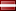###Author Topic: Integer Square Root  (Read 10296 times)

####encryptor256

• Full Member
•• Posts: 250
• Country:• Win64 .##### Integer Square Root
« on: August 11, 2013, 04:02:58 PM »
Hello!

Today i learned one new thing, so, now you have a chance to learn it too.

Integer Square Root.

What is this? - Function, one way of, how to find square root of integer type number.

* You can use this function to determine if your input integer number is squared by some integer number.

This one i found on the internet
and i became so intrigued by it,
and made it available in assembler.

Where i found it, info:
Code: [Select]
`User "A.V.R" on site topic, "How to get a square root. ASSEMBLER?"(http://answers.yahoo.com/question/index?qid=20080920184851AAHPgFS)He says, commented:n^2 = sum of first n odd numbers.e.g., 16 = 4^2 = 1+3+5+7 (first 4 odd numbers)In the early 60s as engineering students with only mechanical adding machines, this is what we used to find square roots.Subtract successive odd numbers from the number, keeping track of the number of numbers subtracted. When the original number decreases to zero the number of odd numbers subtracted equals the square root.`

The basic idea is, subtract odd number sequence until your number gets to zero.

For example, 16, 16 - 1 - 3 - 5 - 7 = 0 => 4x odd numbers, till it gets zero.
For example, 25, 25 - 1 - 3 - 5 - 7 - 9 = 0 => 5x odd numbers, till it gets zero.
For example, 12, 12 - 1 - 3 - 5 - 7 = -4 => Error, below zero, input number does not have integer sq root!!!

And this, following, function "intergerSquareRoot",
returns number on success,
returns zero on failure:

Code: [Select]
`intergerSquareRoot: push ebp mov ebp,esp ; --------------------------------------------------------- %define ebp_number ebp+8 ; --------------------------------------------------------- push ecx push edx ; --------------------------------------------------------- mov ecx,dword 1 mov edx,dword 1 mov eax,dword [ebp_number] ; --------------------------------------------------------- cmp eax,dword 0 je .quitFunction ; --------------------------------------------------------- .loop: cmp eax,ecx jl .loopFailure sub eax,ecx cmp eax,dword 0 je .loopEnd add edx,dword 1 add ecx,dword 2 jmp .loop ; --------------------------------------------------------- .loopFailure: mov eax,dword 0 jmp .quitFunction .loopEnd: mov eax,edx ; --------------------------------------------------------- .quitFunction: ; --------------------------------------------------------- pop edx pop ecx ; --------------------------------------------------------- mov esp,ebp pop ebp ret 4`

Test:

1.
Code: [Select]
`        push dword 81 call intergerSquareRoot        ; EAX now should be 9`
2.
Code: [Select]
`        push dword 2500 call intergerSquareRoot        ; EAX now should be 50`
3.
Code: [Select]
`        push dword 1555 call intergerSquareRoot        ; EAX now should be 0, ERROR, this number does not have integral square root`

So, now you know, one of the things, that might happen, when you press, the square root button, on your calculator.Bye, Encryptor256!
« Last Edit: August 11, 2013, 04:08:56 PM by encryptor256 »
Encryptor256's Investigation \ Research Department.